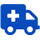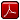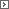Medical MathPrintable Version (pdf)Course Introduction

Core Standards of the Course

Strand 1
Uses of Mathematics in Healthcare

Standard 1
Analyze the use of medical mathematics in the healthcare system

1. Explore different healthcare careers and the math used within the career.
2. Compare and contrast at least two different careers.

Strand 2
Common Mathematical Operations as used in Healthcare

Standard 1
Compute fluently and make reasonable estimates.

1. Evaluate and simplify numerical expressions containing rational numbers using the order of operations.
2. Compute solutions to problems and determine the reasonableness of an answer by relating them to applied scenarios
3. Whole Numbers
• Supplies and inventories
• Intake and output
• Cholesterol
• Quantities
• Nutrition
• Vital signs labs
• Lever systems
• Laboratory Values

Standard 2
Represent real numbers in a variety of ways.

1. Choose appropriate and convenient forms of rational numbers for solving problems and representing answers (e.g., decimal, fraction, or percent).
2. Compute solutions to problems and determine the reasonableness of an answer by relating them to applied scenarios.
3. Decimals
• Tools, instruments
• Nutrition
• Weights
• Rounding
• Temperature
• EKG’s
• Medications
• Laboratory values

Standard 3
Identify relationships among rational numbers and operations involving these numbers.

1. Compute solutions to problems and determine the reasonableness of an answer by relating them to applied scenarios.
2. Fractions
• Tools, instruments
• Weights
• Estimation
• EKG’s
• Medications
• Laboratory values
• Conversions (Fahrenheit/Celsius)

Standard 4
Calculate percentages

1. Compute solutions to problems and determine the reasonableness of an answer by relating them to applied scenarios.
2. Percents
• Chemical solutions
• Laboratory values
• Growth charts
• Medications
• Nutrition
• Intake/output
• Target heart rate
• Stroke volume
• Cardiac output
• Blood loss
• Body surface area, burns
• Oxygen saturation

Strand 3
Ratios and Proportions

Standard 1
Evaluate, solve, and analyze mathematical situations, using algebraic properties and symbols.

1. Solve proportions that include algebraic first-degree expressions (solve for x or use dimensional analysis).
• Nutrition
• Chemical solutions
• Dosage conversions

Standard 2
Use ratios to compare data.

1. Laboratory values
2. Medications
3. Diseases (statistics)analysis).

Strand 4
Gathering Data (Use of Medical Instruments)

Standard 1
Use patterns, relations, and functions to represent mathematical situations.

1. Conversions
2. Metric units
3. Time (12/24)
4. Roman numerals (Arabic/Roman)
5. Temperature (Celsius/Fahrenheit)
6. Pre/Post workout weight analysis
7. Body composition
8. Pharmacology

Standard 2
Represent quantitative relationships using mathematical models and symbols.

1. Find and interpret rates of change by analyzing graphical and numerical data.
2. Understand measureable attributes of objects and the units, systems, and processes of measurement.
3. Solve problems using visualization, and spatial reasoning.
4. Instruments
• IV flow rates
• Macro/micro drops (tubing)
• Syringes
• Rulers, tape measures
• Scales
• Goniometry, ROM
• Centrifuges
• Sphygmomanometer gauges (blood pressure)
• Pulse oximeters
• Oxygen flow rates
• Thermometers

Strand 5
Interpreting Data

Standard 1
Formulate and answer questions by collecting, organizing, and analyzing statistical data.

1. Collect, record, organize, and display a set of statistical data.
2. Determine whether the pattern of the data is linear or nonlinear when given in a list, table, or graph.
3. Interpret the correlation between two variables as positive, negative, or having no correlation.
4. Find a line of best fit by estimation, choosing two points, or using technology for a given set of statistical data.
5. Analyze the meaning of the slope and y-intercept of a line of best fit as it relates to the statistical data set.
6. Find mean, median, mode, and range for a statistical data set.
7. Analyze the meaning of the maximum or minimum and intercepts of the regression equation as they relate to a given set of bivariate data.
8. Analyze the meaning of the maximum or minimum and intercepts of the regression equation as they relate to a given set of bivariate data.
9. Make predictions and estimations and determine their reasonableness using a regression equation (line of best fit).
10. Graphs and charts
• Interpreting charts and graphs
• Temperature, pulse, respirations graphs
• Intake and output charts
• Height, weight, measurement graphics
• Cardiac output
• Medication errors
• Census
• Acuities
• Disease, mortality rates
• Job outlook, projections
• Treatment prognosis
• Clinical trials
• Health care costs
• Effectiveness (facilities, providers)
• Wellness indicators
• Reliability and validity
• Body mass index (BMI)
• Body composition
• Epidemiology

Standard 2
Apply basic concepts of probability.

1. Determine and express the probability of an event as a fraction, percent, ratio, or decimal.
2. Determine the conditional probability of an event (false positive/false negative).

Strand 6
Math for Medications

Standard 1
Compute fluently and make reasonable estimates.

1. Reading drug labels and prescriptions
2. Interpreting prescriptions/Patient instructions

Standard 2
Evaluate, solve, and analyze mathematical situations using algebraic properties and symbols.

1. Simplify and evaluate numerical expressions (including integer exponents and square roots), algebraic expressions, formulas, and equations.
2. Using medical reference books to determine if calculated dosages are safe

Standard 3
Represent quantitative relationships using mathematical models and symbols.

1. Dosing
2. Dosage conversions

Strand 7

Standard 1
Apply systems of order.

1. Numerical filing
2. Appointment scheduling

Standard 2
Evaluate, solve, and analyze mathematical situations using algebraic properties and symbols.

1. Maintaining accounts
2. Checks, deposit slips, and receipts
3. Calculating cash transactions/Payroll
4. Budgeting
5. Depreciation, amortization
6. Insurance

Strand 8
Exponents and Logarithms

Standard 1
Use properties of exponentials to solve equations.

2. Half life

Standard 2
Use properties of logarithms to solve equations.

1. pH

Performance Skills

• Oral presentation on chosen healthcare career mathematics.
• Use healthcare career choice to create a business model.

Workplace Skills

• Critical thinking
• Collaboration
• Communication (Oral/Written)
• Organization
• Technical skills
• Consumer awareness
• Commercial awareness
• Legal requirements/expectations
• Interpersonal relationshipshttp://www.uen.org - in partnership with Utah State Board of Education (USBE) and Utah System of Higher Education (USHE).  Send questions or comments to USBE Specialist - MAREN  HANSEN and see the CTE/Health Science website. For general questions about Utah's Core Standards contact the Director - THALEA  LONGHURST.

These materials have been produced by and for the teachers of the State of Utah. Copies of these materials may be freely reproduced for teacher and classroom use. When distributing these materials, credit should be given to Utah State Board of Education. These materials may not be published, in whole or part, or in any other format, without the written permission of the Utah State Board of Education, 250 East 500 South, PO Box 144200, Salt Lake City, Utah 84114-4200.